$$\newcommand{\id}{\mathrm{id}}$$ $$\newcommand{\Span}{\mathrm{span}}$$ $$\newcommand{\kernel}{\mathrm{null}\,}$$ $$\newcommand{\range}{\mathrm{range}\,}$$ $$\newcommand{\RealPart}{\mathrm{Re}}$$ $$\newcommand{\ImaginaryPart}{\mathrm{Im}}$$ $$\newcommand{\Argument}{\mathrm{Arg}}$$ $$\newcommand{\norm}{\| #1 \|}$$ $$\newcommand{\inner}{\langle #1, #2 \rangle}$$ $$\newcommand{\Span}{\mathrm{span}}$$

# 1.1: Use the Language of Algebra

$$\newcommand{\vecs}{\overset { \rightharpoonup} {\mathbf{#1}} }$$ $$\newcommand{\vecd}{\overset{-\!-\!\rightharpoonup}{\vphantom{a}\smash {#1}}}$$$$\newcommand{\id}{\mathrm{id}}$$ $$\newcommand{\Span}{\mathrm{span}}$$ $$\newcommand{\kernel}{\mathrm{null}\,}$$ $$\newcommand{\range}{\mathrm{range}\,}$$ $$\newcommand{\RealPart}{\mathrm{Re}}$$ $$\newcommand{\ImaginaryPart}{\mathrm{Im}}$$ $$\newcommand{\Argument}{\mathrm{Arg}}$$ $$\newcommand{\norm}{\| #1 \|}$$ $$\newcommand{\inner}{\langle #1, #2 \rangle}$$ $$\newcommand{\Span}{\mathrm{span}}$$ $$\newcommand{\id}{\mathrm{id}}$$ $$\newcommand{\Span}{\mathrm{span}}$$ $$\newcommand{\kernel}{\mathrm{null}\,}$$ $$\newcommand{\range}{\mathrm{range}\,}$$ $$\newcommand{\RealPart}{\mathrm{Re}}$$ $$\newcommand{\ImaginaryPart}{\mathrm{Im}}$$ $$\newcommand{\Argument}{\mathrm{Arg}}$$ $$\newcommand{\norm}{\| #1 \|}$$ $$\newcommand{\inner}{\langle #1, #2 \rangle}$$ $$\newcommand{\Span}{\mathrm{span}}$$

Summary

By the end of this section, you will be able to:

• Find factors, prime factorizations, and least common multiples
• Use variables and algebraic symbols
• Simplify expressions using the order of operations
• Evaluate an expression
• Identify and combine like terms
• Translate an English phrase to an algebraic expression

This chapter is intended to be a brief review of concepts that will be needed in an Intermediate Algebra course. A more thorough introduction to the topics covered in this chapter can be found in the Elementary Algebra chapter, Foundations.

# Find Factors, Prime Factorizations, and Least Common Multiples

The numbers 2, 4, 6, 8, 10, 12 are called multiples of 2. A multiple of 2 can be written as the product of a counting number and 2.Similarly, a multiple of 3 would be the product of a counting number and 3.We could find the multiples of any number by continuing this process.

Counting Number 1 2 3 4 5 6 7 8 9 10 11 12
Multiples of 2 2 4 6 8 10 12 14 16 18 20 22 24
Multiples of 3 3 6 9 12 15 18 21 24 27 30 33 36
Multiples of 4 4 8 12 16 20 24 28 32 36 40 44 48
Multiples of 5 5 10 15 20 25 30 35 40 45 50 55 60
Multiples of 6 6 12 18 24 30 36 42 48 54 60 66 72
Multiples of 7 7 14 21 28 35 42 49 56 63 70 77 84
Multiples of 8 8 16 24 32 40 48 56 64 72 80 88 96
Multiples of 9 9 18 27 36 45 54 63 72 81 90 99 108

MULTIPLE OF A NUMBER

A number is a multiple of $$n$$ if it is the product of a counting number and $$n$$.

Another way to say that 15 is a multiple of 3 is to say that 15 is divisible by 3. That means that when we divide 3 into 15, we get a counting number. In fact, $$\mathrm{15÷3}$$ is 5, so 15 is $$\mathrm{5⋅3}$$.$$\mathrm{15÷3}$$ is 5, so 15 is $$\mathrm{5⋅3}$$.

DIVISIBLE BY A NUMBER

If a number mm is a multiple of n, then m is divisible by n.

If we were to look for patterns in the multiples of the numbers 2 through 9, we would discover the following divisibility tests:

DIVISIBILITY TESTS

A number is divisible by:

• 2 if the last digit is 0, 2, 4, 6, or 8.
• 3 if the sum of the digits is divisible by 3.
• 5 if the last digit is 5 or 0.
• 6 if it is divisible by both 2 and 3.
• 10 if it ends with 0.

example $$\PageIndex{1}$$

Is 5,625 divisible by ⓐ 2? ⓑ 3? ⓒ 5 or 10? ⓓ 6?

$$\text{Is 5,625 divisible by 2?}$$

$$\begin{array}{ll} \text{Does it end in 0, 2, 4, 6 or 8?} & {\text{No.} \\ \text{5,625 is not divisible by 2.}} \end{array}$$ ⓑ

$$\text{5,625 divisible by 3?}$$

$$\begin{array}{ll} {\text{What is the sum of the digits?} \\ \text{Is the sum divisible by 3?}} & {5+6+2+5=18 \\ \text{Yes.} \\ \text{5,625 is divisible by 3.}}\end{array}$$ ⓒ

$$\text{Is 5,625 divisible by 5 or 10?}$$

$$\begin{array}{ll} \text{What is the last digit? It is 5.} & \text{5,625 is divisible by 5 but not by 10.} \end{array}$$ ⓓ

$$\text{Is 5,625 divisible by 6?}$$

$$\begin{array}{ll}\text{Is it divisible by both 2 and 3?} & {\text{No, 5,625 is not divisible by 2, so 5,625 is} \\ \text{not divisible by 6.}} \end{array}$$

example $$\PageIndex{2}$$

Is 4,962 divisible by ⓐ 2? ⓑ 3? ⓒ 5? ⓓ 6? ⓔ 10?

ⓐ yes ⓑ yes ⓒ no ⓓ yes ⓔ no

example $$\PageIndex{3}$$

Is 3,765 divisible by ⓐ 2? ⓑ 3? ⓒ 5? ⓓ 6? ⓔ 10?

ⓐ no ⓑ yes ⓒ yes ⓓ no ⓔ no

In mathematics, there are often several ways to talk about the same ideas. So far, we’ve seen that if m is a multiple of n, we can say that m is divisible by n. For example, since 72 is a multiple of 8, we say 72 is divisible by 8. Since 72 is a multiple of 9, we say 72 is divisible by 9. We can express this still another way.

Since $$\mathrm{8·9=72}$$, we say that 8 and 9 are factors of 72. When we write $$\mathrm{72=8·9}$$, we say we have factored 72.mathrm{8·9=72}\), we say that 8 and 9 are factors of 72. When we write $$\mathrm{72=8·9}$$, we say we have factored 72.Other ways to factor 72 are $$\mathrm{1·72, \; 2·36, \; 3·24, \; 4·18,}$$ and $$\mathrm{6⋅12}$$. The number 72 has many factors: $$\mathrm{1,2,3,4,6,8,9,12,18,24,36,}$$ and $$\mathrm{72}$$.$$mathrm{1·72, \; 2·36, \; 3·24, \; 4·18,}$$ and $$\mathrm{6⋅12}$$. The number 72 has many factors: $$\mathrm{1,2,3,4,6,8,9,12,18,24,36,}$$ and $$\mathrm{72}$$.

FACTORS

If $$\mathrm{a·b=m}$$, then mathrm{a·b=m}\), then a and b are factors of m.

Some numbers, such as 72, have many factors. Other numbers have only two factors. A prime number is a counting number greater than 1 whose only factors are 1 and itself.

PRIME NUMBER AND COMPOSITE NUMBER

A prime number is a counting number greater than 1 whose only factors are 1 and the number itself.

A composite number is a counting number that is not prime. A composite number has factors other than 1 and the number itself.

The counting numbers from 2 to 20 are listed in the table with their factors. Make sure to agree with the “prime” or “composite” label for each!The prime numbers less than 20 are 2, 3, 5, 7, 11, 13, 17, and 19. Notice that the only even prime number is 2.

A composite number can be written as a unique product of primes. This is called the prime factorization of the number. Finding the prime factorization of a composite number will be useful in many topics in this course.

PRIME FACTORIZATION

The prime factorization of a number is the product of prime numbers that equals the number.

To find the prime factorization of a composite number, find any two factors of the number and use them to create two branches. If a factor is prime, that branch is complete. Circle that prime. Otherwise it is easy to lose track of the prime numbers.

If the factor is not prime, find two factors of the number and continue the process. Once all the branches have circled primes at the end, the factorization is complete. The composite number can now be written as a product of prime numbers.

example $$\PageIndex{4}$$: How to Find the Prime Factorization of a Composite Number

Factor 48.We say $$\mathrm{2⋅2⋅2⋅2⋅3}$$ is the prime factorization of 48. We generally write the primes in ascending order. Be sure to multiply the factors to verify your answer.$$\mathrm{2⋅2⋅2⋅2⋅3}$$ is the prime factorization of 48. We generally write the primes in ascending order. Be sure to multiply the factors to verify your answer.

If we first factored 48 in a different way, for example as $$\mathrm{6·8}$$, the result would still be the same. Finish the prime factorization and verify this for yourself.

example $$\PageIndex{5}$$

Find the prime factorization of $$\mathrm{80}$$.

$$\mathrm{2⋅2⋅2⋅2⋅5}$$

example $$\PageIndex{6}$$

Find the prime factorization of $$\mathrm{60}$$.

$$\mathrm{2⋅2⋅3⋅5}$$

FIND THE PRIME FACTORIZATION OF A COMPOSITE NUMBER

1. Find two factors whose product is the given number, and use these numbers to create two branches.
2. If a factor is prime, that branch is complete. Circle the prime, like a leaf on the tree.
3. If a factor is not prime, write it as the product of two factors and continue the process.
4. Write the composite number as the product of all the circled primes.

One of the reasons we look at primes is to use these techniques to find the least common multiple of two numbers. This will be useful when we add and subtract fractions with different denominators.

LEAST COMMON MULTIPLE

The least common multiple (LCM) of two numbers is the smallest number that is a multiple of both numbers.

To find the least common multiple of two numbers we will use the Prime Factors Method. Let’s find the LCM of 12 and 18 using their prime factors.

example $$\PageIndex{7}$$: How to Find the Least Common Multiple Using the Prime Factors Method

Find the least common multiple (LCM) of 12 and 18 using the prime factors method.Notice that the prime factors of 12 $$\mathrm{(2·2·3)}$$ and the prime factors of 18 $$\mathrm{(2⋅3⋅3)}$$ are included in the LCM $$\mathrm{(2·2·3·3)}$$. So 36 is the least common multiple of 12 and 18.

By matching up the common primes, each common prime factor is used only once. This way you are sure that 36 is the least common multiple.

example $$\PageIndex{8}$$

Find the LCM of 9 and 12 using the Prime Factors Method.

36

example $$\PageIndex{9}$$

Find the LCM of 18 and 24 using the Prime Factors Method.

72

FIND THE LEAST COMMON MULTIPLE USING THE PRIME FACTORS METHOD

1. Write each number as a product of primes.
2. List the primes of each number. Match primes vertically when possible.
3. Bring down the columns.
4. Multiply the factors.

# Use Variables and Algebraic Symbols

In algebra, we use a letter of the alphabet to represent a number whose value may change. We call this a variable and letters commonly used for variables are $$x,y,a,b,c.$$

VARIABLE

A variable is a letter that represents a number whose value may change.

A number whose value always remains the same is called a constant.

CONSTANT

A constant is a number whose value always stays the same.

To write algebraically, we need some operation symbols as well as numbers and variables. There are several types of symbols we will be using. There are four basic arithmetic operations: addition, subtraction, multiplication, and division. We’ll list the symbols used to indicate these operations below.

OPERATION SYMBOLS

Operation Notation Say: The result is…
Addition $$a+b$$ $$a$$ plus $$b$$ the sum of $$a$$ and $$b$$
Subtraction $$a−b$$ $$a$$ minus $$b$$ the difference of $$a$$ and $$b$$
Multiplication $$a⋅b,ab,(a)(b),(a)b,a(b)$$ $$a$$ times $$b$$ the product of $$a$$ and $$b$$
Division $$a÷b,\space a/b,\space\frac{a}{b},\space b \overline{\smash{)}a}$$ $$a$$ divided by $$b$$ the quotient of $$a$$ and $$b$$;
$$a$$ is called the dividend, and $$b$$ is called the divisor

When two quantities have the same value, we say they are equal and connect them with an equal sign.

EQUALITY SYMBOL

$$a=b$$ is read “a is equal to b.”

The symbol “$$=$$” is called the equal sign.

On the number line, the numbers get larger as they go from left to right. The number line can be used to explain the symbols “$$<$$” and “$$>$$”.

INEQUALITYThe expressions $$a<b$$ or $$a>b$$ can be read from left to right or right to left, though in English we usually read from left to right. In general,

$a<b \text{ is equivalent to }b>a. \text{For example, } 7<11 \text{ is equivalent to }11>7.$

$a>b \text{ is equivalent to }b<a. \text{For example, } 17>4 \text{ is equivalent to }4<17.$

INEQUALITY SYMBOLS

Inequality Symbols Words
$$a\neq b$$ a is not equal to b.
$$a<b$$ a is less than b.
$$a\leq b$$ a is less than or equal to b.
$$a>b$$ a is greater than b.
$$a\geq b$$ a is greater than or equal to b.

Grouping symbols in algebra are much like the commas, colons, and other punctuation marks in English. They help identify an expression, which can be made up of number, a variable, or a combination of numbers and variables using operation symbols. We will introduce three types of grouping symbols now.

GROUPING SYMBOLS

$\begin{array}{lc} \text{Parentheses} & \mathrm{()} \\ \text{Brackets} & \mathrm{[]} \\ \text{Braces} & \mathrm{ \{ \} } \end{array}$

Here are some examples of expressions that include grouping symbols. We will simplify expressions like these later in this section.

$\mathrm{8(14−8) \; \; \; \; \; \; \; \; 21−3[2+4(9−8)] \; \; \; \; \; \; \; \; 24÷ \{13−2[1(6−5)+4]\}}$

What is the difference in English between a phrase and a sentence? A phrase expresses a single thought that is incomplete by itself, but a sentence makes a complete statement. A sentence has a subject and a verb. In algebra, we have expressions and equations.

EXPRESSION

An expression is a number, a variable, or a combination of numbers and variables using operation symbols.

$\begin{array}{lll} \textbf{Expression} & \textbf{Words} & \textbf{English Phrase} \\ \mathrm{3+5} & \text{3 plus 5} & \text{the sum of three and five} \\ \mathrm{n−1} & n\text{ minus one} & \text{the difference of } n \text{ and one} \\ \mathrm{6·7} & \text{6 times 7} & \text{the product of six and seven} \\ \frac{x}{y} & x \text{ divided by }y & \text{the quotient of }x \text{ and }y \end{array}$

Notice that the English phrases do not form a complete sentence because the phrase does not have a verb.

An equation is two expressions linked by an equal sign. When you read the words the symbols represent in an equation, you have a complete sentence in English. The equal sign gives the verb.

EQUATION

An equation is two expressions connected by an equal sign.

$\begin{array}{ll} \textbf{Equation} & \textbf{English Sentence} \\ 3+5=8 & \text{The sum of three and five is equal to eight.} \\ n−1=14 & n \text{ minus one equals fourteen.} \\ 6·7=42 & \text{The product of six and seven is equal to forty-two.} \\ x=53 & x \text{ is equal to fifty-three.} \\ y+9=2y−3 & y \text{ plus nine is equal to two } y \text{ minus three.} \end{array}$

Suppose we need to multiply 2 nine times. We could write this as $$\mathrm{2·2·2·2·2·2·2·2·2}$$. This is tedious and it can be hard to keep track of all those 2s, so we use exponents. We write $$\mathrm{2·2·2}$$ as $$\mathrm{2^3}$$ and $$\mathrm{2·2·2·2·2·2·2·2·2}$$ as $$\mathrm{2^9}$$. In expressions such as $$\mathrm{2^3}$$, the 2 is called the base and the 3 is called the exponent. The exponent tells us how many times we need to multiply the base.EXPONENTIAL NOTATION

We say $$\mathrm{2^3}$$ is in exponential notation and $$\mathrm{2·2·2}$$ is in expanded notation.

$$a^n$$ means multiply a by itself, n times.The expression $$a^n$$ is read a to the $$n^{th}$$ power.

While we read $$a^n$$ as $$“a$$ to the $$n^{th}$$ power”, we usually read:

$\begin{array}{cc} a^2 & “a \text{ squared}” \\ a^3 & “a \text{ cubed}” \end{array}$

We’ll see later why $$a^2$$ and $$a^3$$ have special names.

Table shows how we read some expressions with exponents.

Expression In Words
72 7 to the second power or 7 squared
53 5 to the third power or 5 cubed
94 9 to the fourth power
125 12 to the fifth power

# Simplify Expressions Using the Order of Operations

To simplify an expression means to do all the math possible. For example, to simplify $$\mathrm{4·2+1}$$ we would first multiply $$\mathrm{4⋅2}$$ to get 8 and then add the 1 to get 9. A good habit to develop is to work down the page, writing each step of the process below the previous step. The example just described would look like this:

$\mathrm{ 4⋅2+1} \\ \mathrm{8+1} \\ \mathrm{9}$

By not using an equal sign when you simplify an expression, you may avoid confusing expressions with equations.

SIMPLIFY AN EXPRESSION

To simplify an expression, do all operations in the expression.

We’ve introduced most of the symbols and notation used in algebra, but now we need to clarify the order of operations. Otherwise, expressions may have different meanings, and they may result in different values.

For example, consider the expression $$\mathrm{4+3⋅7}$$. Some students simplify this getting 49, by adding $$\mathrm{4+3}$$ and then multiplying that result by 7. Others get 25, by multiplying $$\mathrm{3·7}$$ first and then adding 4.

The same expression should give the same result. So mathematicians established some guidelines that are called the order of operations.

USE THE ORDER OF OPERATIONS.

1. Parentheses and Other Grouping Symbols
• Simplify all expressions inside the parentheses or other grouping symbols, working on the innermost parentheses first.
2. Exponents
• Simplify all expressions with exponents.
3. Multiplication and Division
• Perform all multiplication and division in order from left to right. These operations have equal priority.
• Perform all addition and subtraction in order from left to right. These operations have equal priority.

Students often ask, “How will I remember the order?” Here is a way to help you remember: Take the first letter of each key word and substitute the silly phrase “Please Excuse My Dear Aunt Sally”.

$\begin{array}{ll} \text{Parentheses} & \text{Please} \\ \text{Exponents} & \text{Excuse} \\ \text{Multiplication Division} & \text{My Dear} \\ \text{Addition Subtraction} & \text{Aunt Sally} \end{array}$

It’s good that “My Dear” goes together, as this reminds us that multiplication and division have equal priority. We do not always do multiplication before division or always do division before multiplication. We do them in order from left to right.

Similarly, “Aunt Sally” goes together and so reminds us that addition and subtraction also have equal priority and we do them in order from left to right.

example $$\PageIndex{10}$$

Simplify: $$\mathrm{18÷6+4(5−2)}$$.Parentheses? Yes, subtract first.Exponents? No. Multiplication or division? Yes. Divide first because we multiply and divide left to right.Any other multiplication or division? Yes. Multiply.Any other multiplication of division? No. Any addition or subtraction? Yes. Add.example $$\PageIndex{11}$$

Simplify: $$\mathrm{30÷5+10(3−2).}$$

16

example $$\PageIndex{12}$$

Simplify: $$\mathrm{70÷10+4(6−2).}$$

23

When there are multiple grouping symbols, we simplify the innermost parentheses first and work outward.

example $$\PageIndex{13}$$

Simplify: $$\mathrm{5+23+3[6−3(4−2)].}$$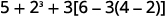Are there any parentheses (or other grouping symbols)? Yes.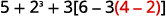Focus on the parentheses that are inside the brackets. Subtract.Continue inside the brackets and multiply.Continue inside the brackets and subtract.The expression inside the brackets requires no further simplification. Are there any exponents? Yes. Simplify exponents.Is there any multiplication or division? Yes. Multiply.Is there any addition of subtraction? Yes. Add.Add.example $$\PageIndex{14}$$

Simplify: $$\mathrm{9+53−[4(9+3)].}$$

86

example $$\PageIndex{15}$$

Simplify: $$\mathrm{72−2[4(5+1)].}$$

1

### Evaluate an Expression

In the last few examples, we simplified expressions using the order of operations. Now we’ll evaluate some expressions—again following the order of operations. To evaluate an expression means to find the value of the expression when the variable is replaced by a given number.

EVALUATE AN EXPRESSION

To evaluate an expression means to find the value of the expression when the variable is replaced by a given number.

To evaluate an expression, substitute that number for the variable in the expression and then simplify the expression.

Example $$\PageIndex{16}$$

Evaluate when $$x=4$$: ⓐ $$x^2$$ ⓑ $$3^x$$ ⓒ $$2x^2+3x+8$$.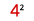Use definition of exponent.Simplify.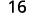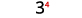Use definition of exponent.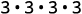Simplify.Follow the order of operations.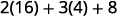example $$\PageIndex{17}$$

Evaluate when $$x=3$$, ⓐ $$x^2$$ ⓑ $$4^x$$ ⓒ $$3x^2+4x+1$$.

ⓐ 9 ⓑ 64 ⓒ40

example $$\PageIndex{18}$$

Evaluate when $$x=6$$, ⓐ $$x^3$$ ⓑ $$2^x$$ ⓒ $$6x^2−4x−7$$.

ⓐ 216 ⓑ 64 ⓒ 185

#### Identify and Combine Like Terms

Algebraic expressions are made up of terms. A term is a constant, or the product of a constant and one or more variables.

TERM

A term is a constant or the product of a constant and one or more variables.

Examples of terms are $$7,y,5x^2,9a,$$ and $$b^5$$.

The constant that multiplies the variable is called the coefficient.

COEFFICIENT

The coefficient of a term is the constant that multiplies the variable in a term.

Think of the coefficient as the number in front of the variable. The coefficient of the term $$3x$$ is 3. When we write $$x$$, the coefficient is 1, since $$x=1⋅x$$.

Some terms share common traits. When two terms are constants or have the same variable and exponent, we say they are like terms.

Look at the following 6 terms. Which ones seem to have traits in common?

$5x \; \; \; 7 \; \; \; n^2 \; \; \; 4 \; \; \; 3x \; \; \; 9n^2$

We say,

$$7$$ and $$4$$ are like terms.

$$5x$$ and $$3x$$ are like terms.

$$n^2$$ and $$9n^2$$ are like terms.

LIKE TERMS

Terms that are either constants or have the same variables raised to the same powers are called like terms.

If there are like terms in an expression, you can simplify the expression by combining the like terms. We add the coefficients and keep the same variable.

$\begin{array}{lc} \text{Simplify.} & 4x+7x+x \\ \text{Add the coefficients.} & 12x \end{array}$

ExAMPLE $$\PageIndex{19}$$: How To Combine Like Terms

Simplify: $$2x^2+3x+7+x^2+4x+5$$.ExAMPLE $$\PageIndex{20}$$

Simplify: $$3x^2+7x+9+7x^2+9x+8$$.

$$10x^2+16x+17$$

ExAMPLE $$\PageIndex{21}$$

Simplify: $$4y^2+5y+2+8y2+4y+5.$$

$$12y^2+9y+7$$

COMBINE LIKE TERMS.

1. Identify like terms.
2. Rearrange the expression so like terms are together.
3. Add or subtract the coefficients and keep the same variable for each group of like terms.

#### Translate an English Phrase to an Algebraic Expression

We listed many operation symbols that are used in algebra. Now, we will use them to translate English phrases into algebraic expressions. The symbols and variables we’ve talked about will help us do that. Table summarizes them.

Operation Phrase Expression

the sum of a and b

a increased by b

b more than a

the total of a and b

$$a+b$$
Subtraction a minus b

the difference of a and b

a decreased by b

b less than a

b subtracted from a

$$a−b$$
Multiplication a times b

the product of aa and bb

twice a

$$a·b,ab,a(b),(a)(b)$$

$$2a$$

Division a divided by b

the quotient of a and b

the ratio of a and b

b divided into a

$$a÷b,a/b,\frac{a}{b},b \overline{\smash{)}a}$$

Look closely at these phrases using the four operations:Each phrase tells us to operate on two numbers. Look for the words of and and to find the numbers.

Example $$\PageIndex{22}$$

Each phrase tells us to operate on two numbers. Look for the words of and and to find the numbers.

Translate each English phrase into an algebraic expression:

ⓐ the difference of $$14x$$ and 9

ⓑ the quotient of $$8y^2$$ and 3

ⓒ twelve more than $$y$$

ⓓ seven less than $$49x^2$$

ⓐ The key word is difference, which tells us the operation is subtraction. Look for the words of and and to find the numbers to subtract.ⓑ The key word is quotient, which tells us the operation is division.ⓒ The key words are more than. They tell us the operation is addition. More than means “added to.”

$\text{twelve more than }y \\ \text{twelve added to }y \\ y+12$

ⓓ The key words are less than. They tell us to subtract. Less than means “subtracted from.”

$\text{seven less than }49x^2 \\ \text{seven subtracted from }49x^2 \\ 49x^2−7$

Exercise $$\PageIndex{23}$$

Translate the English phrase into an algebraic expression:

ⓐ the difference of $$14x^2$$ and 13

ⓑ the quotient of $$12x$$ and 2

ⓒ 13 more than $$z$$

ⓓ 18 less than $$8x$$

ⓐ $$14x^2−13$$ ⓑ $$12x÷2$$

ⓒ $$z+13$$ ⓓ $$8x−18$$

Example $$\PageIndex{24}$$

Translate the English phrase into an algebraic expression:

ⓐ the sum of $$17y^2$$ and 19

ⓑ the product of 7 and y

ⓒ Eleven more than $$x$$

ⓓ Fourteen less than 11a

ⓐ $$17y^2+19$$ ⓑ $$7y$$

ⓒ $$x+11$$ ⓓ $$11a−14$$

We look carefully at the words to help us distinguish between multiplying a sum and adding a product.

Example $$\PageIndex{25}$$

Translate the English phrase into an algebraic expression:

ⓐ eight times the sum of x and y

ⓑ the sum of eight times x and y

There are two operation words—times tells us to multiply and sum tells us to add.

ⓐ Because we are multiplying 8 times the sum, we need parentheses around the sum of x and y, $$(x+y)$$. This forces us to determine the sum first. (Remember the order of operations.)

$\text{eight times the sum of }x \text{ and }y \\ 8(x+y)$

ⓑ To take a sum, we look for the words of and and to see what is being added. Here we are taking the sum of eight times x and y.Example $$\PageIndex{26}$$

Translate the English phrase into an algebraic expression:

ⓐ four times the sum of p and q

ⓑ the sum of four times p and q

ⓐ $$4(p+q)$$ ⓑ $$4p+q$$

Example $$\PageIndex{27}$$

Translate the English phrase into an algebraic expression:

ⓐ the difference of two times x and 8

ⓑ two times the difference of x and 8

ⓐ $$2x−8$$ ⓑ$$2(x−8)$$

Later in this course, we’ll apply our skills in algebra to solving applications. The first step will be to translate an English phrase to an algebraic expression. We’ll see how to do this in the next two examples.

Example $$\PageIndex{28}$$

The length of a rectangle is 14 less than the width. Let w represent the width of the rectangle. Write an expression for the length of the rectangle.

$\begin{array}{lc} \text{Write a phrase about the length of the rectangle.} & \text{14 less than the width} \\ \text{Substitute }w \text{ for “the width.”} & w \\ \text{Rewrite less than as subtracted from.} & \text{14 subtracted from } w \\ \text{Translate the phrase into algebra.} & w−14 \end{array}$

Example $$\PageIndex{29}$$

The length of a rectangle is 7 less than the width. Let w represent the width of the rectangle. Write an expression for the length of the rectangle.

$$w−7$$

Example $$\PageIndex{30}$$

The width of a rectangle is 6 less than the length. Let l represent the length of the rectangle. Write an expression for the width of the rectangle.

$$l−6$$

The expressions in the next example will be used in the typical coin mixture problems we will see soon.

Example $$\PageIndex{31}$$

June has dimes and quarters in her purse. The number of dimes is seven less than four times the number of quarters. Let q represent the number of quarters. Write an expression for the number of dimes.

$\begin{array}{lc} \text{Write a phrase about the number of dimes.} & \text{7 less than 4 times }q \\ \text{Translate 4 times }q. & \text{7 less than 4}q \\ \text{Translate the phrase into algebra.} & 4q−7 \end{array}$

Example $$\PageIndex{32}$$

Geoffrey has dimes and quarters in his pocket. The number of dimes is eight less than four times the number of quarters. Let q represent the number of quarters. Write an expression for the number of dimes.

$$4q−8$$

Example $$\PageIndex{33}$$

Lauren has dimes and nickels in her purse. The number of dimes is three more than seven times the number of nickels. Let n represent the number of nickels. Write an expression for the number of dimes.

$$7n+3$$

# Key Concepts

• Divisibility Tests
A number is divisible by:
2 if the last digit is 0, 2, 4, 6, or 8.
3 if the sum of the digits is divisible by 3.
5 if the last digit is 5 or 0.
6 if it is divisible by both 2 and 3.
10 if it ends with 0.
• How to find the prime factorization of a composite number.
1. Find two factors whose product is the given number, and use these numbers to create two branches.
2. If a factor is prime, that branch is complete. Circle the prime, like a bud on the tree.
3. If a factor is not prime, write it as the product of two factors and continue the process.
4. Write the composite number as the product of all the circled primes.
• How To Find the least common multiple using the prime factors method.
1. Write each number as a product of primes.
2. List the primes of each number. Match primes vertically when possible.
3. Bring down the columns.
4. Multiply the factors.
• Equality Symbol
$$a=b$$ is read “a is equal to b.” The symbol “=” is called the equal sign.
• Inequality• Inequality Symbols
Inequality Symbols Words
$$a≠b$$ a is not equal to b.
$$a<b$$ a is less than b.
$$a≤b$$ a is less than or equal to b.
$$a>b$$ a is greater than b.
$$a≥b$$ a is greater than or equal to b.
• Grouping Symbols $$\begin{array}{lc} \text{Parentheses} & \mathrm{()} \\ \text{Brackets} & \mathrm{[]} \\ \text{Braces} & \mathrm{ \{ \} } \end{array}$$
• Exponential Notation $$a^n$$ means multiply a by itself, n times. The expression an is read a to the $$n^{th}$$ power.
• Simplify an Expression
To simplify an expression, do all operations in the expression.
• How to use the order of operations.
1. Parentheses and Other Grouping Symbols
• Simplify all expressions inside the parentheses or other grouping symbols, working on the innermost parentheses first.
2. Exponents
• Simplify all expressions with exponents.
3. Multiplication and Division
• Perform all multiplication and division in order from left to right. These operations have equal priority.
• Perform all addition and subtraction in order from left to right. These operations have equal priority.
• How to combine like terms.
1. Identify like terms.
2. Rearrange the expression so like terms are together.
3. Add or subtract the coefficients and keep the same variable for each group of like terms.
Operation Phrase Expression
the sum of aa and b
a increased by b
b more than a
the total of a and b
$$a+b$$
Subtraction a minus b
the difference of a and b
a decreased by b
b less than a
b subtracted from a
$$a−b$$
Multiplication a times b
the product of aa and bb
twice a

$$a·b,ab,a(b),(a)(b)$$

$$2a$$

Division

a divided by b

the quotient of a and b

the ratio of a and b

b divided into a
$$a÷b,a/b,\frac{a}{b},b \overline{\smash{)}a}$$

## Practice Makes Perfect

Identify Multiples and Factors

In the following exercises, use the divisibility tests to determine whether each number is divisible by 2, by 3, by 5, by 6, and by 10.utoNum" template (preferably at the end) to the page.

84

[Show Solution]

Divisible by 2, 3, 6

96

896

[Show Solution]

Divisible by 2

942

22,335

[Show Solution]

Divisible by 3, 5

39,075

Find Prime Factorizations and Least Common Multiples

In the following exercises, find the prime factorization.

86

[Show Solution]

$$2⋅43$$

78

455

[Show Solution]

$$5⋅7⋅13$$

400

432

[Show Solution]

$$2⋅2⋅2⋅2⋅3⋅3⋅3$$

627

In the following exercises, find the least common multiple of each pair of numbers using the prime factors method.

8, 12

[Show Solution]

24

12, 16

28, 40

[Show Solution]

420

84, 90

55, 88

[Show Solution]

440

60, 72

Simplify Expressions Using the Order of Operations

In the following exercises, simplify each expression.

$$2^3−12÷(9−5)$$

[Show Solution]

5

$$3^2−18÷(11−5)$$

$$2+8(6+1)$$

[Show Solution]

58

$$4+6(3+6)$$

$$20÷4+6(5−1)$$

[Show Solution]

29

$$33÷3+4(7−2)$$

$$3(1+9⋅6)−4^2$$

[Show Solution]

149

$$5(2+8⋅4)−7^2$$

$$2[1+3(10−2)]$$

[Show Solution]

50

$$5[2+4(3−2)]$$

$$8+2[7−2(5−3)]−3^2$$

[Show Solution]

5

$$10+3[6−2(4−2)]−2^4$$

Evaluate an Expression

In the following exercises, evaluate the following expressions.

When $$x=2$$,

ⓐ $$x^6$$

ⓑ $$4^x$$

ⓒ $$2x^2+3x−7$$

[Show Solution]

ⓐ 64 ⓑ 16 ⓒ 7

When $$x=3$$,

ⓐ $$x^5$$

ⓑ $$5x$$

ⓒ $$3x^2−4x−8$$

When $$x=4,y=1$$

$$x^2+3xy−7y^2$$

[Show Solution]

21

When $$x=3,y=2$$

$$6x^2+3xy−9y^2$$

When $$x=10,y=7$$

$$(x−y)^2$$

[Show Solution]

9

When $$a=3,b=8$$

$$a^2+b^2$$

Simplify Expressions by Combining Like Terms

In the following exercises, simplify the following expressions by combining like terms.

$$7x+2+3x+4$$

[Show Solution]

$$10x+6$$

$$8y+5+2y−4$$

$$10a+7+5a−2+7a−4$$

[Show Solution]

$$22a+1$$

$$7c+4+6c−3+9c−1$$

$$3x^2+12x+11+14x^2+8x+5$$

[Show Solution]

$$17x^2+20x+16$$17x^2+20x+16\)

$$5b^2+9b+10+2b^2+3b−4$$

Translate an English Phrase to an Algebraic Expression

In the following exercises, translate the phrases into algebraic expressions.

ⓐ the difference of $$5x^2$$ and $$6xy$$

ⓑ the quotient of $$6y^2$$ and $$5x$$

ⓒ Twenty-one more than $$y^2$$

ⓓ $$6x$$ less than $$81x^2$$

[Show Solution]

ⓐ $$5x^2−6xy$$ ⓑ $$\frac{6y^2}{5x}$$

ⓒ $$y^2+21$$ ⓓ $$81x^2−6x$$

ⓐ the difference of $$17x^2$$ and $$17x^2$$ and $$5xy$$

ⓑ the quotient of $$8y^3$$ and $$3x$$

ⓒ Eighteen more than $$a^2$$;

ⓓ$$11b$$ less than $$100b^2$$

ⓐ the sum of $$4ab^2$$ and $$3a^2b$$

ⓑ the product of $$4y^2$$ and $$5x$$

ⓒ Fifteen more than $$m$$

ⓓ $$9x$$ less than $$121x^2$$

[Show Solution]

ⓐ $$4ab^2+3a^2b$$ ⓑ $$20xy^2$$

ⓒ $$m+15$$ ⓓ $$121x^2−9x$$ $$9x<121x^2$$

ⓐ the sum of $$3x^2y$$ and $$7xy^2$$

ⓑ the product of $$6xy^2$$ and $$4z$$

ⓒ Twelve more than $$3x^2$$

ⓓ $$7x^2$$ less than $$63x^3$$

ⓐ eight times the difference of $$y$$ and nine

ⓑ the difference of eight times $$y$$ and 9

[Show Solution]

ⓐ $$8(y−9)$$ ⓑ $$8y−9$$

ⓐ seven times the difference of $$y$$ and one

ⓑ the difference of seven times $$y$$ and 1

ⓐ five times the sum of $$3x$$ and $$y$$

ⓑ the sum of five times $$3x$$ and $$y$$

[Show Solution]

ⓐ $$5(3x+y)$$ ⓑ $$15x+y$$

ⓐ eleven times the sum of $$4x2$$ and $$5x$$

ⓑ the sum of eleven times $$4x^2$$ and $$5x$$

Eric has rock and country songs on his playlist. The number of rock songs is 14 more than twice the number of country songs. Let c represent the number of country songs. Write an expression for the number of rock songs.

[Show Solution]

$$14>2c$$

The number of women in a Statistics class is 8 more than twice the number of men. Let $$m$$ represent the number of men. Write an expression for the number of women.

Greg has nickels and pennies in his pocket. The number of pennies is seven less than three the number of nickels. Let n represent the number of nickels. Write an expression for the number of pennies.

[Show Solution]

$$3n-7$$

Jeannette has $$5$$ and $$10$$ bills in her wallet. The number of fives is three more than six times the number of tens. Let $$t$$ represent the number of tens. Write an expression for the number of fives.

## Writing Exercises

Explain in your own words how to find the prime factorization of a composite number.

Why is it important to use the order of operations to simplify an expression?

Explain how you identify the like terms in the expression $$8a^2+4a+9−a^2−1.$$

Explain the difference between the phrases “4 times the sum of x and y” and “the sum of 4 times x and y”.

## Self Check

ⓐ Use this checklist to evaluate your mastery of the objectives of this section.ⓑ If most of your checks were:

…confidently. Congratulations! You have achieved the objectives in this section. Reflect on the study skills you used so that you can continue to use them. What did you do to become confident of your ability to do these things? Be specific.

…with some help. This must be addressed quickly because topics you do not master become potholes in your road to success. In math every topic builds upon previous work. It is important to make sure you have a strong foundation before you move on. Who can you ask for help? Your fellow classmates and instructor are good resources. Is there a place on campus where math tutors are available? Can your study skills be improved?

…no - I don’t get it! This is a warning sign and you must not ignore it. You should get help right away or you will quickly be overwhelmed. See your instructor as soon as you can to discuss your situation. Together you can come up with a plan to get you the help you need.

## Glossary

coefficient
The coefficient of a term is the constant that multiplies the variable in a term.
composite number
A composite number is a counting number that is not prime. It has factors other than 1 and the number itself.
constant
A constant is a number whose value always stays the same.
divisible by a number
If a number m is a multiple of n, then m is divisible by n.
equation
An equation is two expressions connected by an equal sign.
evaluate an expression
To evaluate an expression means to find the value of the expression when the variables are replaced by a given number.
expression
An expression is a number, a variable, or a combination of numbers and variables using operation symbols.
factors
If $$a·b=m$$, then a and b are factors of m.
least common multiple
The least common multiple (LCM) of two numbers is the smallest number that is a multiple of both numbers.
like terms
Terms that are either constants or have the same variables raised to the same powers are called like terms.
multiple of a number
A number is a multiple of n if it is the product of a counting number and n.
order of operations
The order of operations are established guidelines for simplifying an expression.
prime factorization
The prime factorization of a number is the product of prime numbers that equals the number.
prime number
A prime number is a counting number greater than 1 whose only factors are 1 and the number itself.
simplify an expression
To simplify an expression means to do all the math possible.
term
A term is a constant, or the product of a constant and one or more variables.
variable
A variable is a letter that represents a number whose value may change.